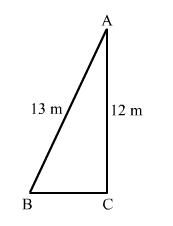# A 13 m long ladder reaches a window of a building 12 m above the ground.Question:

A 13 m long ladder reaches a window of a building 12 m above the ground. Determine the distance of the foot of the ladder from the building.

Solution:

Let AB and AC be the ladder and height of the building.
It is given that:
AB = 13 m and AC = 12 m
We need to find the distance of the foot of the ladder from the building, i.e, BC.
In right-angled triangle ABC, we have:$A B^{2}=A C^{2}+B C^{2}$

$\Rightarrow B C=\sqrt{13^{2}-12^{2}}$

$=\sqrt{169-144}$

$=\sqrt{25}$

$=5 \mathrm{~m}$

Hence, the distance of the foot of the ladder from the building is 5 m